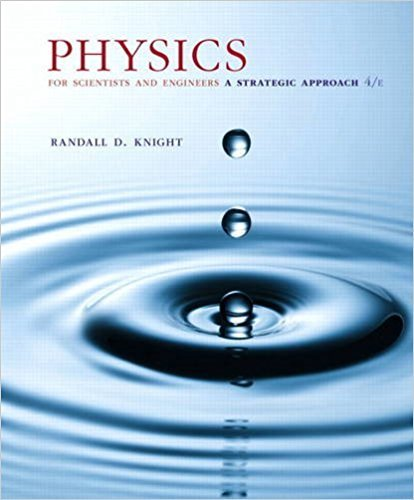×
×

# Solutions for Chapter 13: Newtons Theory of Gravity## Full solutions for Physics for Scientists and Engineers: A Strategic Approach, Standard Edition (Chs 1-36) | 4th Edition

ISBN: 9780134081496Solutions for Chapter 13: Newtons Theory of Gravity

Solutions for Chapter 13
4 5 0 343 Reviews
17
0
##### ISBN: 9780134081496

Chapter 13: Newtons Theory of Gravity includes 99 full step-by-step solutions. Since 99 problems in chapter 13: Newtons Theory of Gravity have been answered, more than 136628 students have viewed full step-by-step solutions from this chapter. This expansive textbook survival guide covers the following chapters and their solutions. Physics for Scientists and Engineers: A Strategic Approach, Standard Edition (Chs 1-36) was written by and is associated to the ISBN: 9780134081496. This textbook survival guide was created for the textbook: Physics for Scientists and Engineers: A Strategic Approach, Standard Edition (Chs 1-36), edition: 4.

Key Physics Terms and definitions covered in this textbook
• //

parallel

• any symbol

average (indicated by a bar over a symbol—e.g., v¯ is average velocity)

• °C

Celsius degree

• °F

Fahrenheit degree

×